# Chapter 11: Absorption And Ion Exchange¶

## Ex11.1: Page 575¶

In :
# Illustration 11.1
# Page: 575

print'Illustration 11.1 - Page: 575\n\n'

# Solution
import numpy
import matplotlib.pyplot as plt
%matplotlib inline
#*****Data*****#
Temp = 30.0;# [OC]
#*************#

# From Fig. 11.5 (Pg 572)
# The isosteres for various concentrations are straight and their slopes are measured with the help of milimeter rule.
# Data = [X(kg acetone/kg carbon) lambda(slope of isostere)]
Data = numpy.array([[0.05 ,1.170],[0.10, 1.245],[0.15 ,1.3],[0.20 ,1.310],[0.25 ,1.340],[0.30 ,1.327]]);# [kg acetone/kg carbon]
lambdar = 551.0;# [reference at 30 OC,kJ/kg]
Val = numpy.zeros(shape=(6,5));
for i in range(0,6):
Val[i,0] = Data[i,0];# [kg acetone/kg carbon]
Val[i,1] = Data[i,1];# [slope of isostere]
Val[i,2] = -Data[i,1]*lambdar;# [kJ/kg acetone]

plt.plot(Val[:,0],Val[:,2])
plt.grid();
xlabel("X (kg carbon / kg acetone)");
ylabel("Differential heat of adsorption (kJ / kg acetone)");
title("Graphical Integration");
plt.show()
# Area: The area under the curve between X = 0 to X = X
# Corresponding to Data(:,1):
Area = numpy.array([-29.8 ,-63.0, -97.9 ,-134.0, -170.5, -207.5]);
for i in range(0,6):
Val[i,3] = Area[i];
Val[i,4] = Area[i]+(lambdar*Val[i,0]);
print " (1) = X(kg acetone/kg carbon) \n (2)= Slope of isostere \n (3)= Differential heat of adsorption(kJ/kg acetone) \n (4)=deltaH_prime(vapour(kJ/kg carbon)) \n (5)=deltaH(liquid(kJ/kg carbon)"
print"(1) \t \t \t \t (2)  \t \t \t  \t (3) \t \t \t \t \t \t \t \t (4) \t \t \t \t \t \t (5) "
for i in range(0,6):
print Val[i,0]," \t \t \t ",Val[i,1]," \t \t ",Val[i,2]," \t \t \t \t \t ",Val[i,3]," \t \t \t \t",Val[i,4]
#the answers are slightly different in textbook due to approximation while here answers are precise

Illustration 11.1 - Page: 575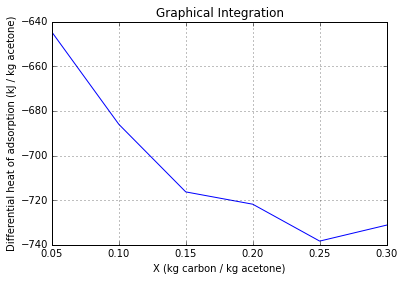(1) = X(kg acetone/kg carbon)
(2)= Slope of isostere
(3)= Differential heat of adsorption(kJ/kg acetone)
(4)=deltaH_prime(vapour(kJ/kg carbon))
(5)=deltaH(liquid(kJ/kg carbon)
(1) 	 	 	 	 (2)  	 	 	  	 (3) 	 	 	 	 	 	 	 	 (4) 	 	 	 	 	 	 (5)
0.05  	 	 	  1.17  	 	  -644.67  	 	 	 	 	  -29.8  	 	 	 	-2.25
0.1  	 	 	  1.245  	 	  -685.995  	 	 	 	 	  -63.0  	 	 	 	-7.9
0.15  	 	 	  1.3  	 	  -716.3  	 	 	 	 	  -97.9  	 	 	 	-15.25
0.2  	 	 	  1.31  	 	  -721.81  	 	 	 	 	  -134.0  	 	 	 	-23.8
0.25  	 	 	  1.34  	 	  -738.34  	 	 	 	 	  -170.5  	 	 	 	-32.75
0.3  	 	 	  1.327  	 	  -731.177  	 	 	 	 	  -207.5  	 	 	 	-42.2


## Ex11.2: Page 596¶

In :
# Illustration 11.2
# Page: 596

print'Illustration 11.2 - Page: 596\n\n'

# solution
import numpy
import matplotlib.pyplot as plt
%matplotlib inline
#*****Data*****#
# x:kg carbon/kg soln
# y_star: Equilibrium colour, units/kg soln.
# X:adsorbate concentration, units/kg carbon
# Data = [x Y_star]
Data =numpy.array([[0, 9.6],[0.001, 8.6],[0.004 ,6.3],[0.008, 4.3],[0.02 ,1.7],[0.04, 0.7]]);
Yo = 9.6;# [units of colour/kg soln]
Y1 = 0.1*Yo;# [units of colour/kg soln]
Ls = 1000.0;# [kg soln]
#****************#

n = 1.66;# [slope of line]
# At X = 663, Y_star = 4.3
# From eqn. 11.5
X = 663;
Y_star = 4.3;
m = Y_star/X**n;
# Freundlich Equation:
def f76(X):
return m*X**n
X = numpy.arange(0,1000,1);

plt.plot(X,f76(X));
plt.grid('on');
plt.xlabel("units of colour/kg carbon");
plt.ylabel("units of colour/kg solution");
title("Equilibium Data(on arithmetic scale)");
plt.show()
# Single Stage Operation:
# Since fresh carbn is used:
Xo = 0;# [units/kg carbon]
# From scf(30):
X1 = 270;# [units/kg carbon]
Data2 =numpy.array([[Xo, Yo],[X1, Y1]]);

plt.plot(X,f76(X),label="Equilbrium curve")
plt.plot(Data2[:,0],Data2[:,1],label="Operating line curve")
plt.grid('on');
plt.xlabel("units of colour/kg carbon");
plt.ylabel("units of colour/kg solution");
plt.legend(loc='upper left');
plt.title("Single stage operation");
plt.show()
# From Eqn. 11.4:
Ss = Ls*((Yo-Y1)/(X1-Xo));# [kg carbon/kg soln]
print"Quantity of fresh carbon recquired for single stage operation: ",Ss," kg carbon/1000 kg solution\n"

# Two stage cross current operation:
# For the minimumamount of carbon:
X1 = 565;# [units/kg carbon]
Y1 = 3.30;# [units of colour/kg soln]
X2 = 270;# [units/kg carbon]
Y2 = 0.96;# [units of colour/kg soln]
Data3 = numpy.array([[Xo ,Yo],[X1 ,Y1]]);
Data4 = numpy.array([[0 ,Y1],[X2 ,Y2]]);

plt.plot(X,f76(X),label="Equilbrium curve")
plt.plot(Data3[:,0],Data3[:,1],label="First of two Cocurrent")
plt.plot(Data4[:,0],Data4[:,1],label="Second of two Cocurrent")
plt.grid('on');
plt.xlabel("units of colour/kg carbon");
plt.ylabel("units of colour/kg solution");
plt.legend(loc='upper left');
plt.title("Two stage Cross current operation");
plt.show()
# From Eqn. 11.8:
Ss1 = Ls*(Yo-Y1)/(X1-Xo);# [kg]
Ss2 = Ls*(Y1-Y2)/(X2-Xo);# [kg]
Ss = Ss1+Ss2;# [kg]
print"Quantity of fresh carbon recquired for two stage crosscurrent operation: ",Ss," kg carbon/1000 kg solution\n"

# Two Stage counter current operation:
Yo = 9.6;
Y2 = 0.96;
# By trial and error:
XNpPlus1 = 0;
X1 = 675;
Data5 = numpy.array([[X1 ,Yo],[XNpPlus1 ,Y2]]);

plt.plot(X,f76(X),label="Equilbrium curve")
plt.plot(Data5[:,0],Data5[:,1],label="Two stage Counter Current");
plt.grid('on');
plt.xlabel("units of colour/kg carbon");
plt.ylabel("units of colour/kg solution");
plt.legend(loc='upper left');
plt.title("Two stage Counter Current operation");
# By eqn 11.14:
Ss = Ls*(Yo-Y2)/(X1-XNpPlus1);
print"Quantity of fresh carbon recquired for two stage Counter Current operation: ",Ss," kg carbon/1000 kg solution\n"

Illustration 11.2 - Page: 596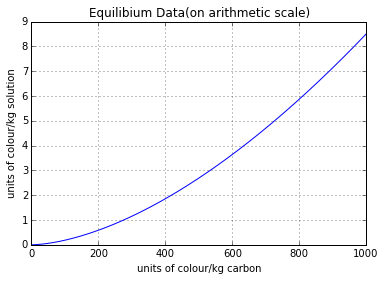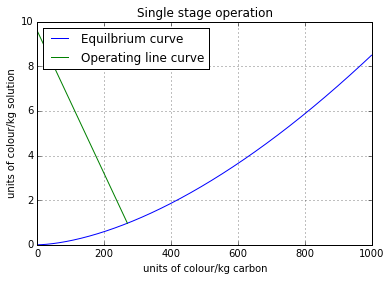Quantity of fresh carbon recquired for single stage operation:  32.0  kg carbon/1000 kg solution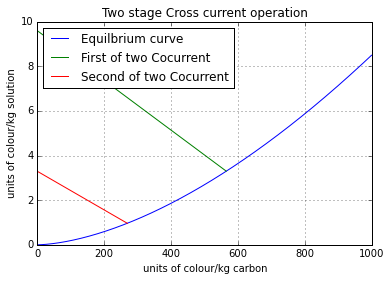Quantity of fresh carbon recquired for two stage crosscurrent operation:  19.8171091445  kg carbon/1000 kg solution

Quantity of fresh carbon recquired for two stage Counter Current operation:  12.8  kg carbon/1000 kg solution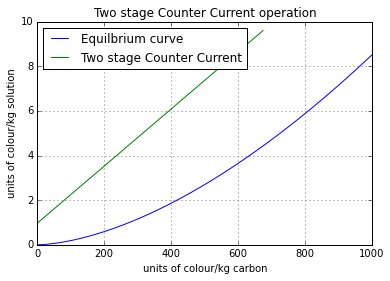## Ex11.3: Page 602¶

In :
# Illustration 11.3
# Page: 602

print'Illustration 11.3 - Page: 602\n\n'

# Solution
import numpy
from scipy.optimize import fsolve
import math
#***Data***#
T = 1.0; #[m]
di = 0.203;# [m]
n = 1;# [for one impeller]
Density_S = 2300.0;# [kg/cubic m]
Density_p = 2300.0;# [kg/cubic m]
C = 0.150;# [m]
S = 50.0;# [kg]
g = 9.807;# [m/s]
dp = 8*10**(-4);# [m]
N = 8.33; #[r/s]
Temp=25;# [OC]
#*************#

# Assume:
Po = 5;
viscosity_L = 8.94*10**(-4);# [kg/m.s]
Density_L = 998.0;# [kg/cubic m]
delta_Density = Density_S-Density_L;# [kg/cubic m]
# By Eqn. 11.23:
Vts = g*dp**2*delta_Density/(18*viscosity_L);# [m/s]
# By defn. of power number:
# P = Po*Density_m*di**5*Ni**3
# vm = math.pi*T**2*(Z+C)/4
# Solid Volume = S/Density_p;
# If these are substituted in Eqn. 11.22
def f(Z):
return (((Z+C)**(1.3/3))*math.exp(4.35*Z/(T-0.1)))-((1.0839*Po*di**(11.0/2)*N**3*Density_p**(2.0/3))/(g*Vts*T**(7.0/6)*S**(2.0/3)))
Z = fsolve(f,7);# [m]
phi_Sm = 4*S/(math.pi*T**2*(Z+C)*Density_p);
Density_m = (phi_Sm*Density_p)+((1-phi_Sm)*Density_L);# [kg/cubic m]
phi_Ss = 0.6;
viscosity_m = viscosity_L/(1-(phi_Sm/phi_Ss))**1.8;# [kg/m.s]
Re = di**2*N*Density_m/viscosity_m;
P = Po*Density_m*N**3*di**5;# [W]
print "Agitator Power required: ",round(P)," W\n"
#the answers are slightly different in textbook due to approximation while here answers are precise

Illustration 11.3 - Page: 602

Agitator Power required:  1113.0  W



## Ex11.4: Page 604¶

In :
# Illustration 11.4
# Page: 604

print'Illustration 11.4 - Page: 604\n\n'

import math
#****Data*****#
# b: kerosene c:water
# c:kg water/cubic m liquid
Density_l = 783;# [kg/cubic m]
viscosity_l = 1.7*10**(-3);# [kg/m.s]
Mb = 200;# [kg/kmol]
Density_p = 881;# [kg/cubic m]
m = 0.522;# [(kg water/cubic m kerosene)/(kg water/kg gel)]
Xo = 0;# [kg H2O/kg gel]
#**************#

# Solution (a)
co = Density_l*4*10**(-5);# [kg water/cubic m]
c1 = Density_l*5*10**(-6);# [kg water/cubic m]
# For Ss minimum:
X1 = c1/m;# [kg H2O/kg gel]
# By Water Balance:
SsminByVl = (co-c1)/(X1-Xo);# [kg gel/cubic m kerosene]
print"Minimum Solid/Liquid ratio used:",SsminByVl," kg gel/cubic m kerosene"
print"\n"

# Solution (b)
# Basis: 1 batch,1.7 cubic m kerosene
Vl = 1.7;# [cubic m]
Ss = 16*1.7;# [kg gel]
V = Ss/Density_p;# [Xol. solid, cubic m]
Vt = 1.7+V;# [Total batch volume, cubic m]
# Take Z = T
T = (Vt*4/math.pi)**(1.0/3);# [m]
# To allow for the  adequate free board:
h = 1.75;# [Vessel height,m]
# Use a six-blade disk impeller.
# From Fig. 11.26:
# dp corresponding to 14 mesh:
dp = 1.4/1000;# [m]
TBydi1 = 2.0;
Value1 = (Density_p-Density_l)/Density_l;
# From Fig. 11.26:
TBydi2 = 4.4;
TBydiAv = (TBydi1+TBydi2)/2.0;
di = T/TBydiAv;# [m]
fr = 0.6;# [settled volume fraction of solids]
Vs = V/fr;# [cubic m]
depth = Vs/((math.pi*(T**2))/4);# [m]
# The depth of settled solid is negligible.
# Locate the turbine 150mm from the bottom of the tank.
C = 0.150;# [m]

# Power:
# Use the sufficient agitator power to lift the solids to 0.6 m above the bottom of the vessel.
Z_prime = 0.6-C;# [m]
# The properties of the slurry in 0.6 m above the bottom of the vessel.
Vm = 0.6*math.pi*T**2.0/4;# [square m]
phi_Sm = V/Vm;# [vol fraction solid]
# From Eqn. 11.24:
Density_m = (phi_Sm*Density_p)+((1-phi_Sm)*Density_l);# [kg/cubic m]
# From Eqn. 11.25:
phi_Ss = 0.8;
viscosity_m = viscosity_l/(1-(phi_Sm/phi_Ss))**1.8;# [kg/m.s]
g = 9.81;# [m/s^2]
# From Eqn. 11.23:
delta_Density = Density_p-Density_l;# [kg/cubic m]
Vts = g*dp**2*delta_Density/(18*viscosity_l);# [m/s]
# From Eqn. 11.22:
n = 1.0;
P = (g*n*Density_m*Vm*Vts)*(phi_Sm**(2.0/3))*(TBydiAv**(1.0/2))*math.exp((4.35*Z_prime/T)-0.1);# [W]
# Assume:
Po = 5.0;
N = (P/(Po*Density_m*di**5))**(1.0/3);# [r/s]
# Use:
N1 = 2.0;# [r/s]
Re = di**2.0*N1*Density_m/viscosity_m;
# From fig. 6.5: Po = 5
# Hence our assumption was right.
print"Power delivered to the slurry: ",round((P*(N1/N)**3),2)," W\n",
print"Power to the motor will be larger, depending on the efficiency of the motor and speed reducer.\n"

# Mass transfer:
# From Eqn. 11.28:
Rep = (dp**(4.0/3))*(P/Vl)**(1.0/3)*(Density_l**(2.0/3)/viscosity_l);
# From Eqn. 2.44:
Temp = 298;# [K]
phi = 1.0;
Va = 0.0756;# [Chapter 2 notation]
Dl = ((117.3*10**(-18))*((phi*Mb)**0.5)*Temp)/(viscosity_l*(Va**(0.6)));
ScL = viscosity_l/(Density_l*Dl);
if  dp<(2.0/1000):
# From Eqn. 11.29:
ShL = 2+(0.47*Rep**0.62*(1/TBydiAv**0.17)*ScL**0.36);
else:
# From Eqn. 11.30:
ShL = 0.222*Rep**(3.0/4)*ScL**(1.0/3);

kL = ShL*Dl/dp;# [m/s]
apS = (math.pi*dp**2)/(math.pi*dp**3*Density_p/6.0);
apL = apS*16;# [square m/cubic m liquid]
Ratio = Ss/(Vl*m);
# From Eqn. 11.40:
thetha = math.log((co/c1)/(1+(1/Ratio)-(1/Ratio)*(co/c1)))/((1+(1/Ratio))*kL*apL);
print"Contacting Time required: ",round(thetha/60,2)," min\n"
#the answers are slightly different in textbook due to approximation while here answers are precise

Illustration 11.4 - Page: 604

Minimum Solid/Liquid ratio used: 3.654  kg gel/cubic m kerosene

Power delivered to the slurry:  350.05  W
Power to the motor will be larger, depending on the efficiency of the motor and speed reducer.

Contacting Time required:  8.3  min



## Ex11.5: Page 606¶

In :
# Illustration 11.5
# Page: 606

print'Illustration 11.5 - Page: 606\n\n'

# Solution

import math
import numpy.linalg as lin
#*****Data******#
Vl = 1.1*10**(-4);# [cubic m/s]
Ss = 0.0012;# [kg/s]
Density_p = 1120;# [kg/cubic m]
dp = 8*10**(-4);# [m]
Ds = 2*10**(-11);# [square m/s]
Dl = 7.3*10**(-10);# [square m/s]
m = 0.2;# [(kg Cu2+/cubic m soln)/(kg Cu2+/kg resin)]
T = 1;# [m]
#********************#

Z = T;# [m]
# The particles will be lifted to the top of the vessel.
Z_prime = 0.5;# [m]
viscosity_l = 8.94*10**(-4);# [kg/m.s]
Density_l = 998;# [kg/cubic m]
delta_Density = Density_p-Density_l;# [kg/cubic m]
g = 9.80;# [m/square s]
# From Eqn. 11.23:
Vts = g*dp**2*delta_Density/(18*viscosity_l);
Vm = math.pi*T**2*Z/4.0;# [cubic m]
Vs = Ss/Density_p;# [cubic m/s]
phi_Sm = Vs/(Vs+Vl);# [vol fraction]
# From eqn. 11.24:
Density_m = (phi_Sm*Density_p)+((1-phi_Sm)*Density_l);# [kg/cubic m]
# From Eqn. 11.22:
n = 1.0;
di = 0.3;# [m]
P = (g*n*Density_m*Vm*Vts)*(phi_Sm**(2.0/3))*((T/di)**(1.0/2))*math.exp((4.35*Z_prime/T)-0.1);# [W]
# To estimate the impeller speed:
# Assume:
Po = 5;
N = (P/(Po*Density_m*di**5))**(1.0/3);# [r/s]
Re = di**2*N*Density_m/viscosity_l;
# From fig. 6.5: Assumption of Po was correct.
print"Speed of the impeller:",round(N,2)," r/s\n"
vT = (math.pi/4.0)*T**2*Z;# [cubic m]
vL = vT*(1-phi_Sm);
# From Eqn. 11.28:
Rep = (dp**(4.0/3))*(P/vL)**(1.0/3)*(Density_l**(2.0/3)/viscosity_l);
ScL = viscosity_l/(Density_l*Dl);
if  dp<(2.0/1000):
# From Eqn. 11.29:
ShL = 2+(0.47*Rep**0.62*((di/T)**0.17)*ScL**0.36);
else:
# From Eqn. 11.30:
ShL = 0.222*Rep**(3.0/4)*ScL**(1.0/3);

ShL = 130.3;# Value wrong in book
kL = ShL*Dl/dp;# [m/s]
# Since the dispersion is uniform throughout the vessel, the residence time for both liquid and solid is same.
thetha = vL*(1-phi_Sm)/Vl;# [s]
# From Fig. 11.27:
abcissa = m*kL*dp/(2*Ds*Density_p);
Parameter = 2*m*kL*thetha/(dp*Density_p);
co = 100*Density_l/10.0**6;# [kg/cubic m]
EMS = 0.63;
Xo = 0;
# From Eqn. 11.44:
# (1): X1-(EMS/m)*c1 = 0
# Solute balance:
# (2): (Ss*X1)+(vL*c1) = (vL*co)+(Xo*Ss)
a = [[1 ,-(EMS/m)],[Ss ,Vl]];
b = [0,(Vl*co)+(Xo*Ss)];
soln =lin.solve(a,b);
X1 = soln;
c1 = soln;
print"Effluent Cu2+ conc. ",round(c1*10**(6)/Density_l,2)," ppm\n",

Illustration 11.5 - Page: 606

Speed of the impeller: 2.71  r/s

Effluent Cu2+ conc.  2.83  ppm


## Ex11.6: Page 616¶

In :
# Illustration 11.6
# Page: 616

print'Illustration 11.6 - Page: 616\n\n'
from scipy.optimize import fsolve
# Solution

#*****Data*****#
# a: air b:silica
Density_a = 1.181;# [kg/cubic m]
Density_b = 671.2;# [kg/cubic m]
kSap = 0.965;# [kg H2O/square m s]
Y1 = 0.005;# [kg H2O/kg dry air]
Y2 = 0.0001;# [kg H2O/kg dry air]
Ss = 0.680;# [square m/s]
Gs = 1.36;# [kg/square m.s]
X2 = 0;# [kg H2O/kg dry air]
# Equilibrium function:
m = 0.0185;
#************#
X1 = (Gs*(Y1-Y2)/Ss)+X2;# [kg H2O/kg dry air]
def  f77(X):
return  m*X
Y2_star = f77(X2);# [kg H2O/kg dry gel]
Y1_star = f77(X1);# [kg H2O/kg dry gel]
deltaY = ((Y1-Y1_star)-(Y2-Y2_star))/math.log((Y1-Y1_star)/(Y2-Y2_star));
NtoG = (Y1-Y2)/deltaY;
# If the fixed bed data are to be used for estimating the mass transfer coeffecient for a moving bed of solids
va = Ss/Density_b;# [m/s]
vb = Gs/Density_a;# [m/s]
rel_v = va+vb;# [relative velocity,m/s]
G_prime = rel_v*Density_a;# [relative mass velocity of air,kg/square m s]
HtG = Gs/(31.6*G_prime**0.55);# [m]
HtS = Ss/kSap;# [m]
# By Eqn. 11.52:
HtoG = HtG+(m*Gs/Ss)*HtS;# [m]
Z = NtoG*HtoG;# [m]
print"Height of continuous countercurrent isothermal absorber for drying: ",round(Z,4)," m\n"

Illustration 11.6 - Page: 616

Height of continuous countercurrent isothermal absorber for drying:  0.2511  m



## Ex11.7: Page 619¶

In :
# Illustration 11.7
# Page: 619

print'Illustration 11.7 - Page: 619\n\n'

# Solution

import numpy.linalg as lin
import matplotlib.pyplot as plt
%matplotlib inline

#*****Data*****#
# a: C2H4 b:C3H8
# The equlibrium curve is plotted in Fig.11.33 (Pg 620)
# C3H8 is more strongly adsorbed component and composition in the gas and adsorbate are expressed as weight fraction C3H8.
Ma = 28;# [kg/kmol]
Mb = 44.1;# [kg/kmol]
xaF = 0.6;# [mole fraction]
xbF = 0.4;# [mole fraction]
xa1 = 0.05;# [mole fraction]
xa2 = 0.95;# [mole fraction]
#***************#

xF = xbF*Mb/((xbF*Mb)+(xaF*Ma));# [wt. fraction C3H8]
xb1 = 1-xa1;# [mole fraction]
x1 = xb1*Mb/((xb1*Mb)+xa1*Ma);# [wt. fraction C3H8]
xb2 = 1-xa2;# [mole fraction]
x2 = xb2*Mb/((xb2*Mb)+(xa2*Ma));# [wt. fraction C3H8]
# Basis: 100 kg feed gas
F = 100.0;# [kg]
# (1): R2+PE = F [From Eqn. 11.63]
# (2): (R2*x2)+(PE*x1) = (F*xF) [From Eqn. 11.64]
# Solving simultaneously:
a = [[1, 1],[x2 ,x1]];
b = [F,(F*xF)];
soln = lin.solve(a,b);
R2 = soln;# [kg]
PE = soln;# [kg]
# Point F at xF and point E1 at x1 are located on the diagram.
# From the diagram:
N1 = 4.57;# [kg carbon/kg adsorbate]
# The minimum reflux ratio is found as it is for the extraction.
delta_Em = 5.80;
Ratio = (delta_Em/N1)-1;# [kg reflux gas/kg product]
R1_m = Ratio*PE;# [kg]
E1_m = R1_m+PE;# [kg]
B_m = N1*E1_m;# [kg carbon/100 kg feed]
Ratio1 = 2*Ratio;
# From Eqn. 11.58:
N_deltaE = (Ratio1+1.0)*N1;# [kg carbon/kg adsorbate]
# Point deltaE is located on the diagram:
R1 = Ratio1*PE;# [kg]
E1 = R1+PE;# [kg]
B = N1*E1;# [kg]
N_deltaR = -(B/R2);# [kg carbon/kg adsorbate]
# Random lines such as the delta_RK are drawn from detaR, and the intersection of equilibrium curves are projected downward in the manner shown to provide the adsorption section operating curve.
# Similarly random lines such as delta_EJ are drawn from deltaE, and the intersections are projected downwards to provide the enriching section operating curve.
# Data = [x x_star]
Data = numpy.array([[0.967 ,0.825],[0.90, 0.710],[0.80 ,0.60],[0.70, 0.50],[0.60 ,0.43],[0.512 ,0.39],[0.40 ,0.193],[0.30, 0.090],[0.20, 0.041],[0.0763, 0.003]]);
Val = zeros(10);
for i in range(0,10):
Val[i] = 1/((Data[i,0])-Data[i,1]);
plt.plot(Data[:,0],Val);
plt.grid('on');
plt.xlabel("x");
plt.ylabel("1 / (x-x*)");
plt.title("Graphical Integraion");
# The area under the curve between x1 & xF, for the enriching section:
Area1 = 2.65;
# The area under the curve between xF & x2, for the adsorption section:
Area2 = 2.67;
r = Ma/Mb;
# From Eqn.11.66:
# For the enriching section:
NtoG1 = Area1-math.log((1+(r-1)*x1)/(1+(r-1)*xF));
# For the adsortion section:
NtoG2 = Area2-math.log((1+(r-1)*x1)/(1+(r-1)*xF));
NtoG = NtoG1+NtoG2;
print"Number of transfer units: ",NtoG

Illustration 11.7 - Page: 619

Number of transfer units:  5.77763695068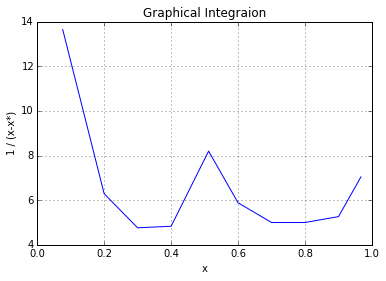## Ex11.8: Page 627¶

In :
# Illustration 11.8
# Page: 627

print'Illustration 11.8 - Page: 627\n\n'

# Solution

from scipy.optimize import fsolve

#******Data******#
rate = 0.1;# [kg/s]
conc = 3.0;# [kg vapour/100cubic m]
Density_p = 720.0;# [kg/cubic m]
Density_bed = 480.0;# [kg/cubic m]
capablity = 0.45;# [kg vapour/kg carbon]
dp = 0.0028;# [m]
time = 3.0;# [h]
#********************#

Vap_adsorbed = time*3600.0*rate;# [kg]
C_required = Vap_adsorbed*1.0/capablity;
# Two beds will be needed: one adsorbing and another regenerated.
totC_required = 2*C_required;# [kg]
print"Amount of carbon required: ",totC_required," kg\n",
Vol = (C_required/Density_bed);
# Assume:
Z = 0.5;# [m]
Area = Vol/Z;# [square m]
# From Eqn. 6.66:
T = 35.0;# [OC]
viscosity_air = 1.82*10**(-5);# [kg/m.s]
Density_air = (29/22.41)*(273.0/(T+273));
e = 1-(Density_bed/Density_p);
G = rate*(100.0/conc)*(Density_air/(Area));# [kg/square m.s]
Re = dp*G/viscosity_air;
Z = 0.5;# [m]
def f78(delta_p):
return ((delta_p/Z)*(e**3*dp*Density_air)/((1-e)*G**2))-(150*(1-e)/Re)-1.75
delta_p = fsolve(f78,7);
print"The pressure drop is:",round(delta_p,2)," N/square m\n"
#the answers are slightly different in textbook due to approximation while here answers are precise

Illustration 11.8 - Page: 627

Amount of caron required:  4800.0  kg
The pressure drop is: 1413.31  N/square m



## Ex11.9: Page 636¶

In :
# Illustration 11.9
# Page: 636

print'Illustration 11.9 - Page: 636\n\n'
import matplotlib.pyplot as plt
%matplotlib inline
# Solution

#*****Data******#
Yo = 0.00267;# [kg H2O/kg dry air]
Yb = 0.0001;# [kg H2O/kg dry air]
Ye = 0.024;# [kg H2O/kg dry air]
Z = 0.61;# [m]
G_prime = 0.1295;# [kg/square m.s]
#******************#

# The equilicrium data is plotted in Fig. 11.45 (Pg 637)
# The gel is initially "dry" and the effluent air initially of so low a humidity asto be substantially dry, so that the operating line passes through the origin of the figure
# The operating line is then drawn to intersect the equilibrium curve.
# Data = [Y[kg H2O/kg dry air] Y_star[kg H2O/kg dry air]]
Data =numpy.array([[0.0001, 0.00003],[0.0002, 0.00007],[0.0004 ,0.00016],[0.0006, 0.00027],[0.0008, 0.00041],[0.0010, 0.00057],[0.0012 ,0.000765],[0.0014, 0.000995],[0.0016, 0.00123],[0.0018 ,0.00148],[0.0020 ,0.00175],[0.0022 ,0.00203],[0.0024 ,0.00230]])
Val1 = zeros(13);
# Val1 = [1/(Y-Y_star)]
for i in range(0,13):
Val1[i] = 1/(Data[i,0]-Data[i,1]);

# Graphical Integration:
plt.plot(Data[:,0],Val1);
plt.grid('on');
plt.xlabel("Y(kg H20 / kg dry air)");
plt.ylabel("1 / (Y-Y_star)");
plt.title("Graphical Integration");
plt.show()
# Area under The curve between Y = Yb and Y = Y:
Area = [0 ,0.100 ,2.219 ,2.930 ,3.487 ,3.976 ,4.438 ,4.915, 5.432, 6.015, 6.728 ,7.716 ,9.304];
# The total number of transfer unit corresponding to adsorption zone:
NtoG = 9.304;
Val2 = zeros(13);
Val3 = zeros(13);
# Val2 = [(w-wb)/wo]
# Val3 = [Y/Yo]
for i in range(0,13):
Val2[i] = Area[i]/NtoG;
Val3[i] = Data[i,0]/Yo;

# Eqn. 11.74 can be arranged as follows:
# f = integrate((1-(Y/Yo)),(w-wb)/wa,0,1)

plt.plot(Val2,Val3);
plt.grid('on');
plt.xlabel("(w-wb) / wo");
plt.ylabel("Y / Yo");
plt.title("Break through curve");
plt.show()
# From area above the curve of scf(2):
f = 0.530;

Gs = G_prime;# [kg/square m.s]
# From Illustration: 11.6
kYap = 31.6*G_prime**0.55;# [kg H2O/cubic m s delta_Y]
kSap = 0.965;# [kg H2O/cubic m s delta_X]
# From Fig. 11.48:
Xt = 0.0858;# [kg H2O/kg gel]
# From Eqn. 11.76:
Ss = Yo*Gs/Xt;# [kg/square m.s]
m = 0.0185;# [average slope of equilibrium curve]
# From Eqn. 11.51 & Eqn. 11.52:
HtG = Gs/kYap;# [m]
HtS = Ss/kSap;# [m]
HtoG = HtG+(m*Gs/Ss)*HtS;# [m]
# From Eqn. 11.79:
Za = NtoG*HtoG;# [m]
# From Eqn. 11.74:
Degree = (Z-(f*Za))/Z;
Density_bed = 671.2;# [Illustration 11.6, kg/cubic m]
mass_gel = Z*Density_bed;# [kg/square m]
# At saturation point the gel contins:
Y1 = mass_gel*Degree*Xt;# [kg H2O/square m cross section]
# The air introduces:
Y2 = Gs*Yo;# [kg/square m s]
print"Time to reach breakpoint is: ",round((Y1/(Y2*3600)),4)," h\n"

Illustration 11.9 - Page: 636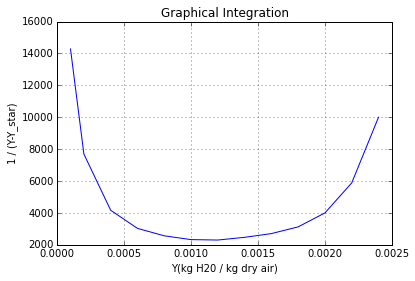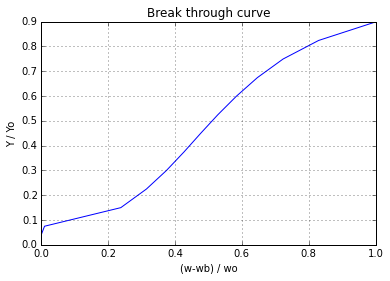Time to reach breakpoint is:  24.7778  h



## Ex11.10: Page 640¶

In :
# Illustration 11.10
# Page: 640

print'Illustration 11.10 - Page: 640\n\n'

# Solution

#*****Data******#
# a:N2 b:H2O
Mb = 18;# [kg/kmol]
Ma = 29;# [kg/kmol]
Z = 0.268;# [m]
Xo_solid = 0.01;# [kg H20/kg solid]
Density_bed = 712.8;# [kg/cubic m]
T = 28.3;# [OC]
P = 593;# [kN/square m]
Gs = 4052;# [kg/square m.h]
Xo_gas = 1440*10**(-6);# [mole fraction]
#********************#

# Yo_star is in equilibrium with Xo:
Xo = 0;# [kg H20/kg solid]
Yo_star = 0;# [kg H20/kg N2]
thetha_t = 12.8;# [h]
thetha_b = 9;# [h]
# The breakthrough data are plotted in the manner of Fig. 11.47 (Pg 639) and thetha_s is dtermined:
thetha_s = 10.9;# [h]
Xt = 0.21;# [kg H20/kg solid]
# From Eqn. 11.81:
LUB = (Z/thetha_s)*(thetha_s-thetha_b);
# For thetha_b = 15 h
thetha_b = 15;# [h]
Yo = (Xo_gas/(1-Xo_gas))*(Mb/Ma);# [kg H20/kg N2]
# From Eq. 11.82:
Zs = Gs*(Yo-Yo_star)*thetha_b/(Density_bed*(Xt-Xo_solid));# [m]
# From Eqn. 11.85:
Z = LUB+Zs;
print"Height of adsorbent column:",round(Z,4)," m\n"

Illustration 11.10 - Page: 640

Height of adsorbent column: 0.0467  m



## Ex11.11: Page 654¶

In :
# Illustration 11.11
# Page: 645

print'Illustration 11.11 - Page: 645\n\n'

# Solution

import math
from scipy.optimize import fsolve
import matplotlib.pyplot as plt
%matplotlib inline

#****Data****#
# For collection of Cu2+:
V = 37850.0;# [l/h]
c1 = 20.0;# [meq Cu2+/l]
c2 = 0.01*c1;# [meq Cu2+/l]
Mass_rate = 2.0;# [meq Cu2+/g resin h (meq Cu2+/l)]
exchanged = V*(c1-c2);# [meq/h]
X2 = 0.30;# [meq Cu2+/g]
#************#/

# The point(c2,X2) is plotted in Fig. 11.48(a), Pg 645:
# For the minimum resin/solution ratio and an infinitely tall tower, the operating line pass though point P.
X = 4.9;# [meq Cu2+/g]
MinRate = exchanged/(X-X2);# [g/h]
Rate = 1.2*MinRate;# [g/h]
# Copper balance:
X1 = (exchanged/Rate)+X2;# [meq Cu2+/g resin]
# The point (c1,x1) is ploted in Fig. 11.48(a) and operating line drawn can be straight line at this low conc.
# Adapting Eqn. 11.48 and rearranging:
# S*Z*Density_s = (V/Mass_rate)*integrate(1/(c-c_star),c,c1,c2)
# Mass_rate = KL_prime*ap/Density_s
# From the equilibrium curve:
# Data = [c c_star]
Data = numpy.array([[20 ,2.4],[16 ,1.9],[12, 0.5],[8 ,0.25],[4 ,0.10],[2 ,0.05],[1 ,0.02],[0.2, 0]]);
Val = zeros(8);
for i in range(0,8):
Val[i] = 1/(Data[i,0]-Data[i,1]);

plt.plot(Data[:,0],Val);
plt.grid('on');
plt.xlabel("c");
plt.ylabel("1 / (c-c*)");
plt.title("Graphical Integration");
# From Graphical Integration:
Area = 5.72;
# holdup = S*Z*Density_s
holdup = V*Area/(Mass_rate);
print"Resin Holdup: ",holdup,"g\n"

# Regeneration of resin:
# For 70% utilisation of 2N acid, feed must contain:
V = exchanged;
F = V/(0.70*2000);# [l/h]
c1 = 0;# [meq Cu2+/l]
c2 = V*1.0/F;# [meq Cu2+/l]
X1 = 0.30;# [meq Cu2+/g resin]
X2 = 4.12;# [meq cu2+/g resin]
# The points (c1,X1) and (c2,X2) are plotted on Fig 11.48(b), Pg 645
c1_star = 120.0;# [meq Cu2+/l]
c2_star = 1700.0;# [meq Cu2+/l]
logmean = ((c1_star-c1)-(c2_star-c2))/math.log((c1_star-c1)/(c2_star-c2));
Mass_rate = 0.018;# [meq Cu2+/g resin h (meq Cu2+/l)]
# Substituting in equation:
def  f79(holdup):
return (V*(c2-c1))-(Mass_rate*holdup*logmean)
holdup = fsolve(f79,7);
print"Resin Holdup in the regeneration Tower is ",round(holdup,3)," g\n"
#the answers are in textbook is wrong

Illustration 11.11 - Page: 645

Resin Holdup:  108251.0 g

Resin Holdup in the regeneration Tower is  296720391.501  g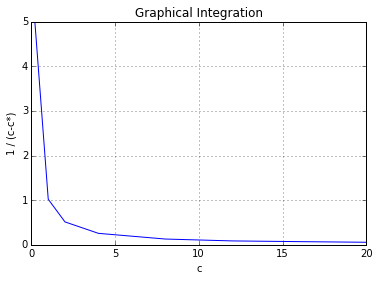In [ ]: